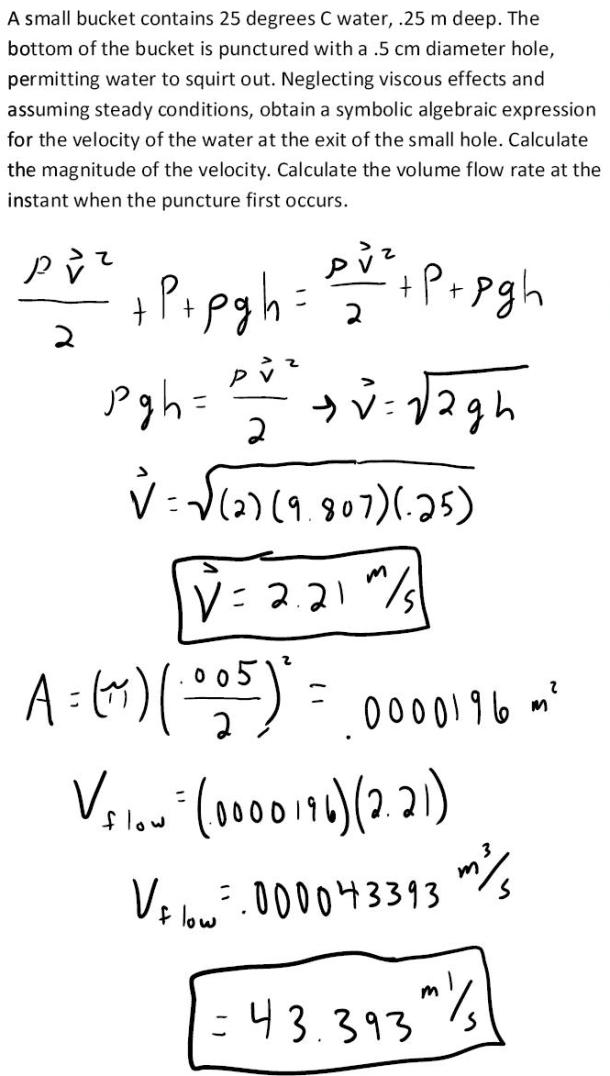fluid mechanics math problems engineering equations fluid mechanics basic mechanics school homework engineering math fluids formulas fluid mechanics problems fluid mechanics problem solutions to fluids problems full solution fluid mechanics engineering fluids problem solution fluid mechanics math problems engineering equations fluid mechanics basic mechanics school homework engineering math fluid mechanics formulas fluid problems fluid mechanics problem solutions to fluid mechanics problems full solution fluid mechanics engineering fluid mechanics problem solution
fluid mechanics math problems engineering equations fluid mechanics basic mechanics school homework engineering math fluids formulas fluid mechanics problems fluid mechanics problem solutions to fluids problems full solution fluid mechanics engineering fluids problem solution fluid mechanics math problems engineering equations fluid mechanics basic mechanics school homework engineering math fluid mechanics formulas fluid problems fluid mechanics problem solutions to fluid mechanics problems full solution fluid mechanics engineering fluid mechanics problem solution
Highalphabet Home Page fluid mechanics solutions fluids math solved Fluid Mechanics Page 1
A small bucket contains 25 degrees C water, .25 m deep. The bottom of the bucket is punctured with a .5 cm diameter hole, permitting water to squirt out. Neglecting viscous effects and assuming steady conditions, obtain a symbolic algebraic expression for the velocity of the water at the exit of the small hole. Calculate the magnitude of the velocity. Calculate the volume flowrate at the instant when the puncture first occurs.A small bucket contains 25 degrees C water, .25 m deep. The bottom of the bucket is punctured with a .5 cm diameter hole, permitting water to squirt out. Neglecting viscous effects and assuming steady conditions, obtain a symbolic algebraic expression for the velocity of the water at the exit of the small hole. Calculate the magnitude of the velocity. Calculate the volume flowrate at the instant when the puncture first occurs.